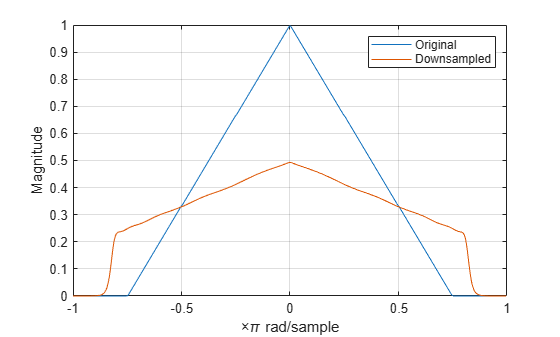## Filtering Before Downsampling

This example shows how to filter before downsampling to mitigate the distortion caused by aliasing. You can use `decimate` or `resample` to filter and downsample with one function. Alternatively, you can lowpass filter your data and then use `downsample`. Create a signal with baseband spectral support greater than $\pi$ radians. Use `decimate` to filter the signal with a 10th-order Chebyshev type I lowpass filter prior to downsampling.

Create the signal and plot the magnitude spectrum.

```F = [0 0.2500 0.5000 0.7500 1.0000]; A = [1.00 0.6667 0.3333 0 0]; Order = 511; B = fir2(Order,F,A); [Hx,W] = freqz(B,1,8192,'whole'); Hx = [Hx(4098:end) ; Hx(1:4097)]; omega = -pi+(2*pi/8192):(2*pi)/8192:pi; plot(omega,abs(Hx)) xlim([-pi pi]) grid title('Magnitude Spectrum') xlabel('Radians/Sample') ylabel('Magnitude')```Filter the signal with a 10th-order type I Chebyshev lowpass filter and downsample by 2. Plot the magnitude spectra of the original signal along with the filtered and downsampled signal.

```y = decimate(B,2,10); [Hy,W] = freqz(y,1,8192,'whole'); Hy = [Hy(4098:end) ; Hy(1:4097)]; hold on plot(omega,abs(Hy),'r','linewidth',2) legend('Original Signal','Downsampled Signal')```The lowpass filter reduces the amount of aliasing distortion outside the interval $\left[-\pi /2,\pi /2\right]$.